Data Structures and Algorithms with Object-Oriented Design Patterns in C#Hash Function Implementations

The preceding section presents methods of hashing integer-valued keys. In reality, we cannot expect that the keys will always be integers. Depending on the application, the keys might be letters, character strings or even more complex data structures such as Associations or Containers.

In general given a set of keys, K, and a positive constant, M, a hash function is a function of the formIn practice is it convenient to implement the hash function h as the composition of two functions f and g. The function f maps keys into integers: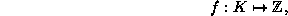where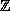is the set of integers. The function g maps non-negative integers into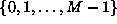: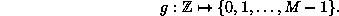Given appropriate functions f and g, the hash function h is simply defined as the composition of those functions: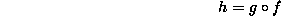That is, the hash value of a key x is given by g(f(x)).

By decomposing the function h in this way, we can separate the problem into two parts: The first involves finding a suitable mapping from the set of keys K to the non-negative integers. The second involves mapping non-negative integers into the interval [0,M-1]. Ideally, the two problems would be unrelated. That is, the choice of the function f would not depend on the choice of g and vice versa. Unfortunately, this is not always the case. However, if we are careful, we can design the functions in such a way that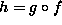is a good hash function.

The hashing methods discussed in the preceding section deal with integer-valued keys. But this is precisely the domain of the function g. Consequently, we have already examined several different alternatives for the function g. On the other hand, the choice of a suitable function for f depends on the characteristics of its domain.

In the following sections, we consider various different domains (sets of keys) and develop suitable hash functions for each of them. Each domain considered corresponds to a C# class. Recall that every C# class is ultimately derived from the System.Object class and that the System.Object class declares a method called GetHashCode:

```namespace System
{
public class object
{
public virtual int GetHashCode () { /* ... */ }
// ...
}
}```
The GetHashCode method corresponds to the function f which maps keys into integers.Copyright © 2001 by Bruno R. Preiss, P.Eng. All rights reserved.